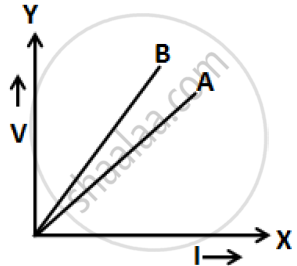# The V-i Graph for a Series Combination and for a Parallel Combination of Two Resistors is Shown in the Figure Below. Which of the Two a Or B. - Physics

The V-I graph for a series combination and for a parallel combination of two resistors is shown in the figure below. Which of the two A or B. represents the parallel combination? Give reasons for your answer.#### Solution 1

For the same change in I, change in V is less for the straight line A than for the straight line B (i.e. the straight line A is less steep than B). The straight line A represents small resistance, while the straight line B represents more resistance. The equivalent resistance is less in a parallel combination than in a series combination. So, line A represents a parallel combination.

#### Solution 2

‘A’ shows parallel combination because in case of parallel combination, the resistance is less for their potential is less, currents is more.

Concept: System of Resistors - Resistors in Series
Is there an error in this question or solution?

#### APPEARS IN

ICSE Class 10 Physics
Chapter 7 Electricity
Figure Based Short Answers | Q 6